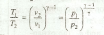# Adiabatic Process or Isentropic Process

A process, in which the working substance neither receives nor gives out heat to its surroundings, during its expansion or compression is called an adiabatic process or isentropic process. This will happen when the working substance remains thermally insulated, so that no heat enters or leaves it during the process. It is thus obvious, that in an adiabatic process:

1. No heat leaves or enters the gas,
2. The temperature of the gas changes, as the work is done at the cost of internal energy, and
3. The change in internal energy is equal to the work done.

We know that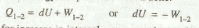Minus sign indicates, that for increase in internal energy, work must be done on the gas (i.e. -ve work must be done by the gas). Similarly, for decrease in internal energy, work must be done by the gas.

* A frictionless adiabatic process is known as Isentropic process (or constant entropy process).

Now consider a certain quantity of a perfect gas being expanded adiabatically which is shown by the curve 1-2 in Fig. 5.4.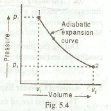Let

v1 = Initial volume of gas,
p 1 = Initial pressure of gas,
v2 = Final volume of gas, and
p2 = Final pressure of gas.

The workdone during adiabatic process is given by

(For expansion)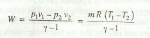(For compression)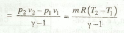Notes: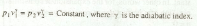(b) Since p1v1 = mRT1 and p2v2 = mRT2, therefore the above equation may be written as